### Home > A2C > Chapter 10 > Lesson 10.2.6 > Problem10-157

10-157.
1. Solve each equation. Homework Help ✎

1.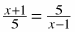2.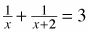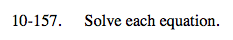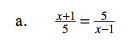Multiply both sides by 5 and x − 1.

x2 − 1 = 25

Solve for x.

x2 = 26

$x = \pm \sqrt{26}$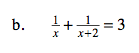See part (a).